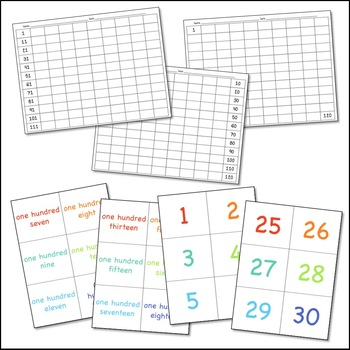Total:
\$0.00# 120's Chart Fill-In Math ActivityK - 5th, Homeschool
Subjects
Standards
Resource Type
Formats Included
• PDF
Pages
48 pages

### Description

Teacher friends,

This simple math activity can be used as a center, math practice or math intervention, for higher grades. Included are number cards and word number cards (all the way from 1-120) for students to pull and read. Once they have read the card, they can fill in one of the 120's charts. There are several 120's charts to choose from, whether students are completely proficient from 1-120 or they need more support.

As a fourth grade teacher, I used this to begin math interventions with my lower mathematicians. It was a great way to open their minds and then use the filled in 100's chart to support their addition and subtraction skills and to help them notice patterns. I hope you enjoy!

Looking for more freebies and math games ? Look no further!:

Thank you,

Kelly Anne

Total Pages
48 pages
N/A
Teaching Duration
N/A
Report this Resource to TpT
Reported resources will be reviewed by our team. Report this resource to let us know if this resource violates TpT’s content guidelines.

### Standards

to see state-specific standards (only available in the US).
Use multiplication and division within 100 to solve word problems in situations involving equal groups, arrays, and measurement quantities, e.g., by using drawings and equations with a symbol for the unknown number to represent the problem.
Interpret a multiplication equation as a comparison, e.g., interpret 35 = 5 × 7 as a statement that 35 is 5 times as many as 7 and 7 times as many as 5. Represent verbal statements of multiplicative comparisons as multiplication equations.
Understand that the three digits of a three-digit number represent amounts of hundreds, tens, and ones; e.g., 706 equals 7 hundreds, 0 tens, and 6 ones. Understand the following as special cases:
100 can be thought of as a bundle of ten tens - called a “hundred.”
The numbers 100, 200, 300, 400, 500, 600, 700, 800, 900 refer to one, two, three, four, five, six, seven, eight, or nine hundreds (and 0 tens and 0 ones).